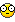# guictrlcreateupdown

## Recommended Posts

```Global \$random,\$pass

#include <GuiConstants.au3>
GuiCreate("", 180, 100,-1, -1 , \$WS_POPUP)
GUiSetBkColor(0x00000)
\$Input_2 = GuiCtrlCreateInput("1", 120, 20, 60, 20)
GUICtrlCreateUpdown(-1,\$UDS_NOTHOUSANDS)
GUICtrlSetLimit(-1,999999,1)
\$Input_1 = GuiCtrlCreateInput(\$pass, 0, 20, 120, 20)
GUICtrlCreateLabel("",0,0,140,20,\$SS_CENTER ,\$GUI_WS_EX_PARENTDRAG)
GUICtrlSetColor(-1,0xffffff)
\$Button_2 = GuiCtrlCreateButton("Copy to clipboard", 00, 40, 180, 20)
\$Button_3 = GuiCtrlCreateButton("Create password", 0, 60, 180, 20)
\$Button_4 = GuiCtrlCreateButton("Exit", 0, 80, 180, 20)
GuiSetState()

do
\$msg = GuiGetMsg()
if \$msg = \$Button_2 then ClipPut(GUICtrlRead(\$Input_1))
if \$msg = \$Button_4 then Exit
if \$msg = \$Button_3 Then passcreate()
until \$msg = \$GUI_EVENT_CLOSE

func passcreate()
GUICtrlSetData(\$Input_1,"")
for \$b = 1 to GUICtrlRead(\$Input_2)
\$random[\$b] = ""
Next
for \$iCC = 1 to  GUICtrlRead(\$Input_2)
if Random(1,3,1) = 1 Then
\$random[\$iCC] = random(48,57,1)
GUICtrlSetData(\$Input_1,\$pass)
Elseif Random(1,3,1) = 2 then
\$random[\$iCC] = random(65,90,1)
GUICtrlSetData(\$Input_1,\$pass)
Else
\$random[\$iCC]= random(97,122,1)
GUICtrlSetData(\$Input_1,\$pass)
EndIf
Next
EndFunc```

for some reason it wont higher and 16959

Edited by Alek

[font="Impact"]Never fear, I is here.[/font]

##### Share on other sites

```Global \$random,\$pass

#include <GuiConstants.au3>
GuiCreate("", 180, 100,-1, -1 , \$WS_POPUP)
GUiSetBkColor(0x00000)
\$Input_2 = GuiCtrlCreateInput("1", 120, 20, 60, 20)
GUICtrlCreateUpdown(-1,\$UDS_NOTHOUSANDS)
GUICtrlSetLimit(-1,999999,1)
\$Input_1 = GuiCtrlCreateInput(\$pass, 0, 20, 120, 20)
GUICtrlCreateLabel("",0,0,140,20,\$SS_CENTER ,\$GUI_WS_EX_PARENTDRAG)
GUICtrlSetColor(-1,0xffffff)
\$Button_2 = GuiCtrlCreateButton("Copy to clipboard", 00, 40, 180, 20)
\$Button_3 = GuiCtrlCreateButton("Create password", 0, 60, 180, 20)
\$Button_4 = GuiCtrlCreateButton("Exit", 0, 80, 180, 20)
GuiSetState()

do
\$msg = GuiGetMsg()
if \$msg = \$Button_2 then ClipPut(GUICtrlRead(\$Input_1))
if \$msg = \$Button_4 then Exit
if \$msg = \$Button_3 Then passcreate()
until \$msg = \$GUI_EVENT_CLOSE

func passcreate()
GUICtrlSetData(\$Input_1,"")
for \$b = 1 to GUICtrlRead(\$Input_2)
\$random[\$b] = ""
Next
for \$iCC = 1 to  GUICtrlRead(\$Input_2)
if Random(1,3,1) = 1 Then
\$random[\$iCC] = random(48,57,1)
GUICtrlSetData(\$Input_1,\$pass)
Elseif Random(1,3,1) = 2 then
\$random[\$iCC] = random(65,90,1)
GUICtrlSetData(\$Input_1,\$pass)
Else
\$random[\$iCC]= random(97,122,1)
GUICtrlSetData(\$Input_1,\$pass)
EndIf
Next
EndFunc```

for some reason it wont higher and 16959

I'm assuming the max value that can be used here is the max size of a signed integer(32,767). I set that as the limit, and it seems to work## Create an account

Register a new account

• ### Recently Browsing   0 members

×

• Wiki

• Back

• #### Beta

• Git
• FAQ
×
• Create New...